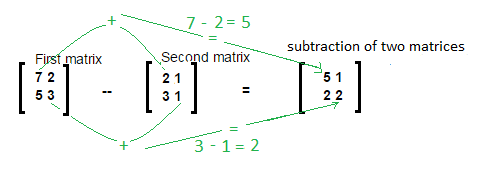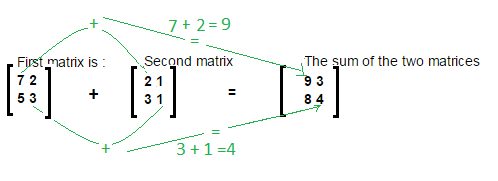### Matrix Subtraction program in java

Hi! we will learn how to add subtract matrices in java.
Both matrices must have same number of rows and columns.

Let’s understand subtraction of matrices by diagram.Main logic behind subtraction is:
//Subtraction of matrices.
int[][] resultMatix = new int[rows][columns];
for (int i = 0; i < rows; i++) {
for (int j = 0; j < columns; j++) {
resultMatix[i][j] = matrix1[i][j] - matrix2[i][j];
}
}

Example/ Full Program/SourceCode to Matrix Subtraction in java >
 package matrix; import java.util.Scanner; /** * @author AnkitMittal */ /** Copyright (c), AnkitMittal  JavaMadeSoEasy.com - Matrix Subtraction in java */ public class MatrixSubtraction {    public static void main(String...args) {           Scanner scanner = new Scanner(System.in);           System.out.print("Enter number of rows in matrix : "); //rows and columns in matrix1 and matrix2 must be same for subtraction.           int rows = scanner.nextInt();           System.out.print("Enter number of columns in matrix : ");           int columns = scanner.nextInt();           int[][] matrix1 = new int[rows][columns];           int[][] matrix2 = new int[rows][columns];                     System.out.println("Enter the elements in first matrix :");           for (int i = 0; i < rows; i++) {                  for (int j = 0; j < columns; j++) {                        matrix1[i][j] = scanner.nextInt();                  }           }           System.out.println("Enter the elements in second matrix :");           for (int i = 0; i < rows; i++) {                  for (int j = 0; j < columns; j++) {                        matrix2[i][j] = scanner.nextInt();                  }           }                     //Subtraction of matrices.           int[][] resultMatix = new int[rows][columns];           for (int i = 0; i < rows; i++) {                  for (int j = 0; j < columns; j++) {                        resultMatix[i][j] = matrix1[i][j] - matrix2[i][j];                  }           }           System.out.println("\nFirst matrix is : ");           for (int i = 0; i < rows; i++) {                  for (int j = 0; j < columns; j++) {                        System.out.print(matrix1[i][j] + " ");                  }                  System.out.println();           }           System.out.println("\nSecond matrix is : ");           for (int i = 0; i < rows; i++) {                  for (int j = 0; j < columns; j++) {                        System.out.print(matrix2[i][j] + " ");                  }                  System.out.println();           }           System.out.println("\nThe subtraction of the two matrices is : ");           for (int i = 0; i < rows; i++) {                  for (int j = 0; j < columns; j++) {                        System.out.print(resultMatix[i][j] + " ");                  }                  System.out.println();           }    } } /*OUTPUT Enter number of rows in matrix : 2 Enter number of columns in matrix : 2 Enter the elements in first matrix : 7 2 5 3 Enter the elements in second matrix : 2 1 3 1 First matrix is : 7 2 5 3 Second matrix is : 2 1 3 1 The subtraction of the two matrices is : 5 1 2 2 */

So we learned program to do Matrix Subtraction in java.

Having any doubt? or you you liked the tutorial! Please comment in below section.

>Matrix Addition in java